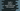# Swift 4 while and repeat-while loop tutorial with exampleIn this tutorial, we will learn about while and repeat-while loops in swift. For both cases, I have added one example :

### 1. While loop :

while loop first checks a condition. If the condition is true, it will run a single or multiple statements defined for the loop. The syntax form of a while loop is :

``````while (condition){
(statements)
}
``````

So, if condition is true, it will enter inside the braces -> execute all statements and then return back to the condition again. This loop will run infinite time if we don’t change the condition checking for each iteration. Below example shows how to print all the elements of a dictionary using while loop :

``````let number = [1:"One" , 2:"Two" , 3:"Three" , 4:"Four" , 5:"Five" , 6:"Six" , 7:"Seven" , 8:"Eight" , 9:"Nine" , 10:"Ten"]

var count = 10

while(count > 0 ){

print (number[count]!)
count -= 1
}
``````

Output :

``````Ten
Nine
Eight
Seven
Six
Five
Four
Three
Two
One
``````

Initial value of count is 10 . First while loop will check if it is greater than 0 or not. If true, it will enter the loop. You can see that we are decrementing the count value by 1 after each iteration. So, the loop will run for count 10,9,8,7,6,5,4,3,2 and 1 .Each time, we will print the value of the dictionary using count as key. After 1 it will become 0 . Since count should be always more than 0 to enter the loop, for count = 0 , it will not run and move to the next step i.e. line after the while loop close braces ’}‘.

### repeat-while loop in swift :

repeat-while is the other variation of while loop. The main difference between while and repeat-while is that for while, the condition is checked first and then the statements inside the curly braces executes. But for repeat-while, first the statements are executed and after that the condition is checked. If the condition is true, control will move to the statements inside curly braces again. Syntax form for ‘repeat-while’ loop is :

``````repeat{
statements
}while (condition)
``````

Let’s try the above example with ‘repeat-while’ :

``````let number = [1:"One" , 2:"Two" , 3:"Three" , 4:"Four" , 5:"Five" , 6:"Six" , 7:"Seven" , 8:"Eight" , 9:"Nine" , 10:"Ten"]

var count = 10

repeat {
print (number[count]!)
count -= 1
}while(count > 0)
``````

Output will be same as above :

``````Ten
Nine
Eight
Seven
Six
Five
Four
Three
Two
One
``````

Basically, it will work same as like ‘while’ loop. First the statement is executed and then condition is checked. print statement will run for count 10,9,8,7,6,5,4,3,2 and 1. Each time it will print out the value from number dictionary by taking count as key.